SLVS776B January   2009  – November 2014

PRODUCTION DATA.

1. Features
2. Applications
3. Description
4. Simplified Schematic
5. Revision History
6. Pin Configuration and Functions
7. Specifications
8. Parameter Measurement Information
9. Detailed Description
1. 9.1 Overview
2. 9.2 Functional Block Diagrams
3. 9.3 Feature Description
4. 9.4 Device Functional Modes
10. 10Applications and Implementation
1. 10.1 Application Information
2. 10.2 Typical Applications
1. 10.2.1 Specific Application, Fixed Output Voltage Supply
1. 10.2.1.1 Design Requirements
2. 10.2.1.2 Detailed Design Procedure
3. 10.2.1.3 Application Curves
2. 10.2.2 Specific Application, Variable Output Voltage Supply
11. 11Power Supply Recommendations
12. 12Layout
13. 13Device and Documentation Support
1. 13.1 Device Support
2. 13.2 Documentation Support
5. 13.5 Electrostatic Discharge Caution
6. 13.6 Glossary
14. 14Mechanical, Packaging, and Orderable Information

• DCK|6
• DCK|6

## 7 Specifications

### 7.1 Absolute Maximum Ratings

over operating free-air temperature range (unless otherwise noted)(1)
TPS6122x UNIT
VIN Input voltage on VIN, L, VOUT, EN, FB –0.3 to 7.5 V
TJ Operating junction temperature –40 to 150 °C
(1) Stresses beyond those listed under Absolute Maximum Ratings may cause permanent damage to the device. These are stress ratings only, and functional operation of the device at these or any other conditions beyond those indicated under Recommended Operating Conditions is not implied. Exposure to absolute-maximum-rated conditions for extended periods may affect device reliability.

### 7.2 Handling Ratings

MIN MAX UNIT
Tstg Storage temperature range –65 150 °C
V(ESD) Electrostatic discharge Human body model (HBM), per ANSI/ESDA/JEDEC JS-001, all pins(1) –2 2 kV
Charged device model (CDM), per JEDEC specification JESD22-C101, all pins(2) –1.5 1.5 kV
(1) JEDEC document JEP155 states that 500V HBM rating allows safe manufacturing with a standard ESD control process.
(2) JEDEC document JEP157 states that 250V CDM rating allows safe manufacturing with a standard ESD control process.

### 7.3 Recommended Operating Conditions

MIN NOM MAX UNIT
VIN Supply voltage at VIN 0.7 5.5 V
TJ Operating virtual junction temperature –40 125 °C

### 7.4 Thermal Information

THERMAL METRIC(1) TPS6122x UNIT
DCK
6 PINS
RθJA Junction-to-ambient thermal resistance 231.2 °C/W
RθJCtop Junction-to-case (top) thermal resistance 61.8
RθJB Junction-to-board thermal resistance 78.8
ψJT Junction-to-top characterization parameter 2.2
ψJB Junction-to-board characterization parameter 78.0
RθJCbot Junction-to-case (bottom) thermal resistance n/a

### 7.5 Electrical Characteristics

over recommended free-air temperature range and over recommended input voltage range (typical at an ambient temperature range of 25°C) (unless otherwise noted)
PARAMETER TEST CONDITIONS MIN TYP MAX UNIT
DC/DC STAGE
VIN Input voltage 0.7 5.5 V
VIN Minimum input voltage at startup RLoad ≥ 150 Ω 0.7 V
VOUT TPS61220 output voltage VIN < VOUT 1.8 6.0 V
VFB TPS61220 feedback voltage 483 500 513 mV
VOUT TPS61221 output voltage (3.3 V) VIN < VOUT 3.20 3.30 3.41 V
VOUT TPS61222 output voltage (5 V) VIN < VOUT 4.82 5.00 5.13 V
ILH Inductor current ripple 200 mA
ISW switch current limit VOUT = 3.3 V, VIN = 1.2 V, TA = 25°C 240 400 mA
VOUT = 3.3 V 200 400 mA
RDSon_HSD Rectifying switch on resistance VOUT = 3.3 V 1000
VOUT = 5.0 V 700
RDSon_LSD Main switch on resistance VOUT = 3.3 V 600
VOUT = 5.0 V 550
Line regulation VIN < VOUT 0.5%
Load regulation VIN < VOUT 0.5%
IQ Quiescent current VIN IO = 0 mA, VEN = VIN = 1.2 V, VOUT = 3.3 V
0.5 0.9 μA
VOUT 5 7.5 μA
ISD Shutdown current VIN VEN = 0 V, VIN = 1.2 V, VOUT ≥ VIN 0.2 0.5 μA
ILKG_VOUT Leakage current into VOUT VEN = 0 V, VIN = 1.2 V, VOUT = 3.3 V 1 μA
ILKG_L Leakage current into L VEN = 0 V, VIN = 1.2 V, VL = 1.2 V, VOUT ≥ VIN 0.01 0.2 μA
IFB TPS61220 Feedback input current VFB = 0.5 V 0.01 μA
IEN EN input current Clamped on GND or VIN (VIN < 1.5 V) 0.005 0.1 μA
CONTROL STAGE
VIL EN input low voltage VIN ≤ 1.5 V 0.2 × VIN V
VIH EN input high voltage VIN ≤ 1.5 V 0.8 × VIN V
VIL EN input low voltage 5 V > VIN > 1.5 V 0.4 V
VIH EN input high voltage 5 V > VIN > 1.5 V 1.2 V
VUVLO Undervoltage lockout threshold for turn off VIN decreasing 0.5 0.7 V
Overvoltage protection threshold 6.0 7.5 V
Overtemperature protection 140 °C
Overtemperature hysteresis 20 °C

### 7.6 Typical Characteristics

TABLE OF GRAPHS FIGURE
Maximum Output Current versus Input Voltage (TPS61220, TPS61221, TPS61222) Figure 1
Efficiency versus Output Current, VOUT = 1.8 V, VIN = [0.7 V; 1.2 V; 1.5 V] (TPS61220) Figure 2
versus Output Current, VIN = [0.7 V; 1.2 V; 2.4 V; 3 V] (TPS61221) Figure 3
versus Output Current, VIN = [0.7 V; 1.2 V; 2.4V; 3.6 V; 4.2 V] (TPS61222) Figure 4
versus Input Voltage, VOUT = 1.8 V, IOUT = [100 µA; 1 mA; 10 mA; 50 mA] (TPS61220) Figure 5
versus Input Voltage, IOUT = [100 µA; 1 mA; 10 mA; 50 mA] (TPS61221) Figure 6
versus Input Voltage, IOUT = [100 µA; 1 mA; 10 mA; 50 mA] (TPS61222) Figure 7
Input Current at No Output Load, Device Enabled (TPS61220, TPS61221, TPS61222) Figure 8
Output Voltage versus Output Current, VOUT = 1.8 V, VIN = [0.7 V; 1.2 V] (TPS61220 ) Figure 9
versus Output Current, VIN = [0.7 V; 1.2 V; 2.4 V] (TPS61221) Figure 10
versus Output Current, VIN = [0.7 V; 1.2 V; 2.4 V; 3.6 V] (TPS61222) Figure 11
versus Input Voltage, Device Disabled, RLOAD = [1 kΩ; 10 kΩ] (TPS6122x) Figure 12
Waveforms Output Voltage Ripple, VIN = 0.8 V, VOUT = 1.8 V, IOUT = 20 mA (TPS61220) Figure 13
Output Voltage Ripple VIN = 1.8 V, IOUT = 50 mA (TPS61221) Figure 14
Load Transient Response, VIN = 1.2 V, IOUT = 6 mA to 50 mA (TPS61221) Figure 15
Load Transient Response, VIN = 2.4 V, IOUT = 14 mA to 126 mA (TPS61222) Figure 16
Line Transient Response, VIN = 1.8 V to 2.4 V, RLOAD = 100 Ω (TPS61221) Figure 17
Line Transient Response, VIN = 2.8 V to 3.6 V, RLOAD = 100 Ω (TPS61222) Figure 18
Startup after Enable, VIN = 0.7 V, VOUT = 1.8 V, RLOAD = 150 Ω (TPS61220) Figure 19
Startup after Enable, VIN = 0.7 V, RLOAD = 150 Ω, (TPS61222) Figure 20
Continuous Current Operation, VIN = 1.2 V, VOUT = 1.8 V, IOUT = 50mA (TPS61220 ) Figure 21
Discontinuous Current Operation, VIN = 1.2 V, VOUT = 1.8 V, IOUT = 10mA (TPS61220) Figure 22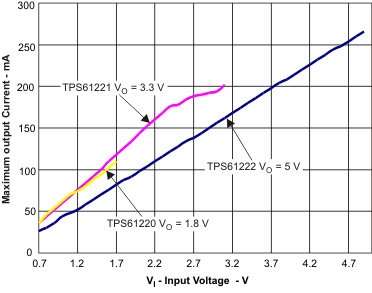Figure 1. Maximum Output Current versus Input Voltage (TPS61220, TPS61221, TPS61222)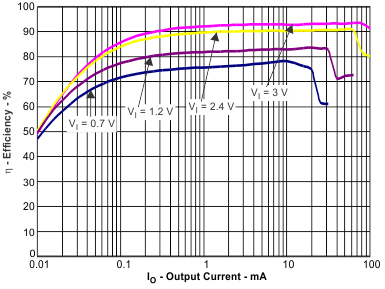VO = 3.3 V
Figure 3. Efficiency versus Output Current and Input Voltage (TPS61221)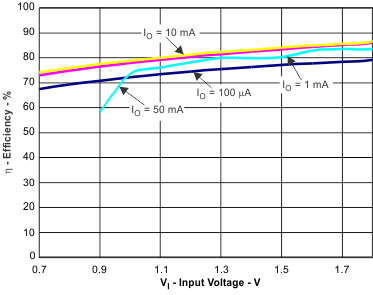VO = 1.8 V
Figure 5. Efficiency versus Input Voltage and Output Current (TPS61220)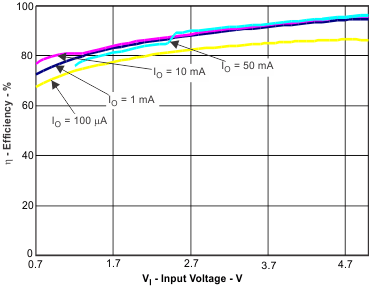VO = 5 V
Figure 7. Efficiency versus Input Voltage and Output Current (TPS61222)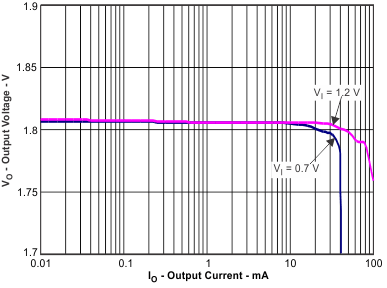VO = 1.8 V
Figure 9. Output Voltage versus Output Current and Input Voltage (TPS61220)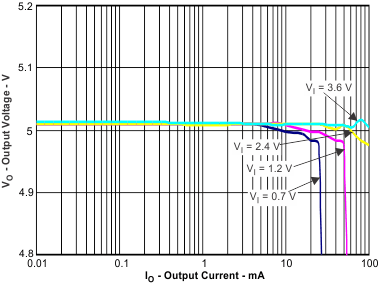VO = 5 V
Figure 11. Output Voltage versus Output Current and Input Voltage (TPS61222)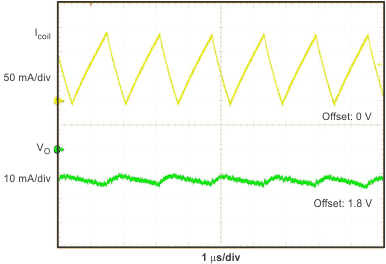VI = 0.8 V VO = 1.8 V IO = 20 mA
Figure 13. Output Voltage Ripple (TPS61220)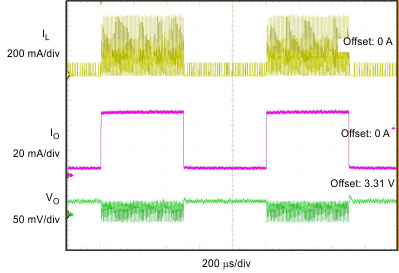VI = 1.2 V IO = 6 mA to 50 mA
Figure 15. Load Transient Response (TPS61221)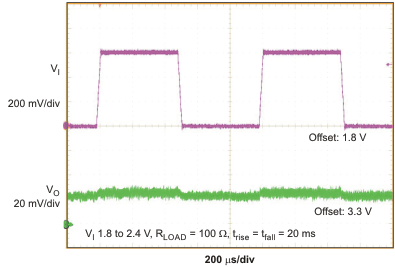VI = 2.4 V to 2.4 V RLOAD = 100 Ω trise = tfall = 20 ms
Figure 17. Line Transient Response (TPS61221)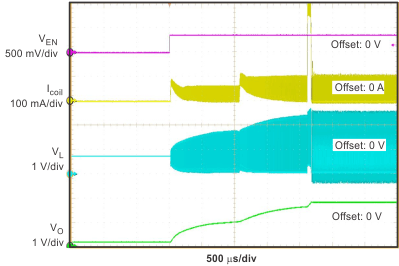VI = 0.7 V VO = 1.8 V RLOAD = 150 Ω
Figure 19. Startup After Enable (TPS61120)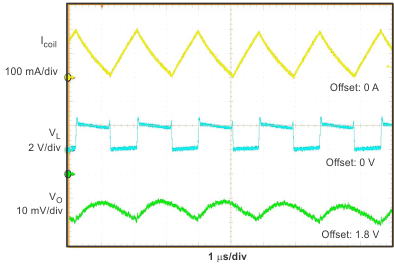VI = 1.2 V VO = 1.8 V IO = 50 mA
Figure 21. Continuous Current Operation (TPS61220)VO = 1.8 V
Figure 2. Efficiency versus Output Current and Input Voltage (TPS61220)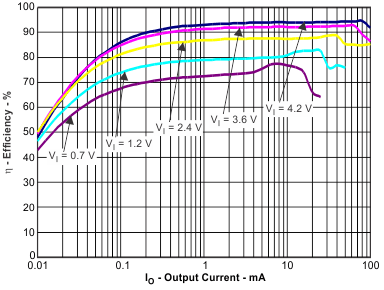VO = 5 V
Figure 4. Efficiency versus Output Current and Input Voltage (TPS61222)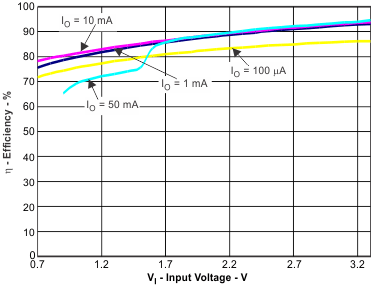VO = 3.3 V
Figure 6. Efficiency versus Input Voltage and Output Current (TPS61221)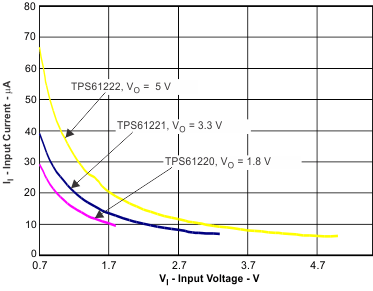Device enabled
Figure 8. No Load Input Current versus Input Voltage, Device Enabled (TPS61220, TPS61221, TPS61222)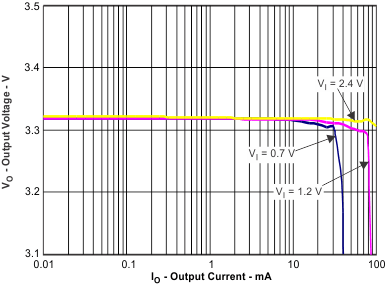VO = 3.3 V
Figure 10. Output Voltage versus Output Current and Input Voltage (TPS61221)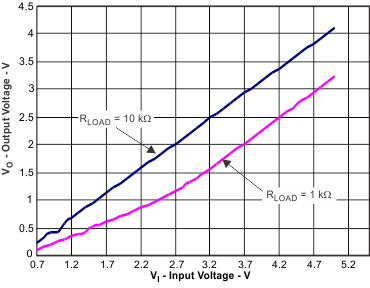VEN = 0 V
Figure 12. Output Voltage versus Input Voltage, Device Disabled (TPS61220)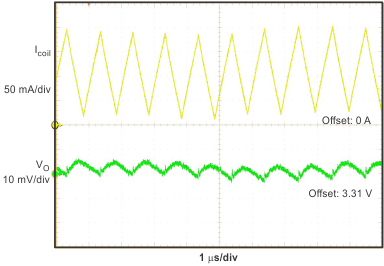VI = 1.8 V VO = 3.3 V IO = 50 mA
Figure 14. Output Voltage Ripple (TPS61221)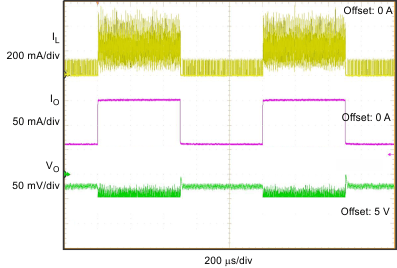VI = 2.4 V IO = 14 mA to 126 mA
Figure 16. Load Transient Response (TPS61222)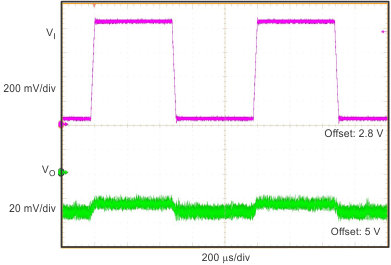VI = 2.8 V to 3.6 V RLOAD = 100 Ω trise = tfall = 20 ms
Figure 18. Line Transient Response (TPS61222)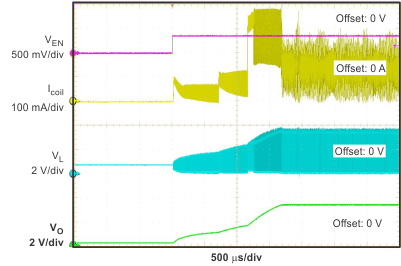VI = 0.7 V VO = 3.3 V RLOAD = 50 Ω
Figure 20. Startup After Enable (TPS61221)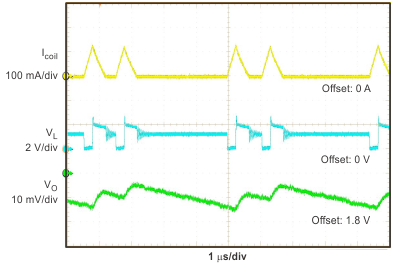VI = 1.2 V VO = 1.8 V IO = 10 mA
Figure 22. Discontinuous Current Operation (TPS61220)